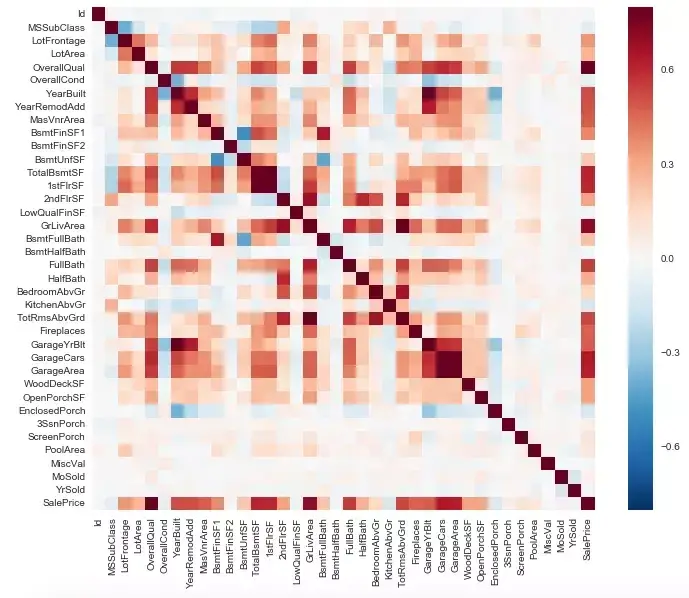• kaggle 是什么
• 如何参赛
• 解决问题一般步骤
• 进一步：
• 如何探索数据
• 如何构造特征

• 提交结果

### kaggle 是什么？

Kaggle 是一个数据科学竞赛的平台，很多公司会发布一些接近真实业务的问题，吸引爱好数据科学的人来一起解决。
https://www.kaggle.com/

https://www.kaggle.com/competitions

### 如何参赛？

Overview： 首先在 overview 中仔细阅读问题的描述，这个比赛是让我们预测房价，它会给我们 79 个影响房价的变量，我们可以通过应用 random forest，gradient boosting 等算法，来对房价进行预测。

Data：在这里给我们提供了 train 数据集，用来训练模型；test 数据集，用来将训练好的模型应用到这上面，进行预测，这个结果也是要提交到系统进行评价的；sample_submission 就是我们最后提交的 csv 文件中，里面的列的格式需要和这里一样。

Kernels：可以看到一些参赛者分享的代码。

Discussion：参赛者们可以在这里提问，分享经验。

### 解决问题一般步骤？

1. 识别问题
2. 探索数据
3. 数据预处理
4. 将 train.csv 分成 train 和 valid 数据
5. 构造新的重要特征数据
6. 应用算法模型
7. 优化模型
8. 选择提取重要特征
9. 再次选择模型，进行训练
10. 调参
11. 重复上述过程，进一步调优
12. 预测

1. 探索数据
2. 特征工程
3. 建立模型
4. 调参
5. 预测提交

http://blog.csdn.net/aliceyangxi1987/article/details/71079448

http://blog.csdn.net/aliceyangxi1987/article/details/71079473

### 如何探索数据？

1. 首先，在 data_description.txt 这里有对 79 个变量含义非常详细的描述

OverallQual: Overall material and finish quality 物料和质量应该是很重要的组成。
GrLivArea: Above grade (ground) living area square feet 面积也是明显的因素。
YearBuilt: Original construction date 时间也有影响。

2. 接着，对要预测的目标数据 y 有一个宏观的把握，这里是输出 summary，也可以用 boxplot，histogram 等形式观察

``````df_train['SalePrice'].describe()

count      1460.000000
mean     180921.195890
std       79442.502883
min       34900.000000
25%      129975.000000
50%      163000.000000
75%      214000.000000
max      755000.000000
Name: SalePrice, dtype: float64``````

count 就是有多少行观察记录，另外注意一下 min 并未有小于 0 的这样的不合理的数值。

3. 通过 Correlation matrix 观察哪些变量会和预测目标关系比较大，哪些变量之间会有较强的关联

``````#correlation matrix
corrmat = df_train.corr()
f, ax = plt.subplots(figsize=(12, 9))
sns.heatmap(corrmat, vmax=.8, square=True);``````

TotalBsmtSF: Total square feet of basement area
1stFlrSF: First Floor square feet

``````#saleprice correlation matrix
k = 10 #number of variables for heatmap
cols = corrmat.nlargest(k, 'SalePrice')['SalePrice'].index
cm = np.corrcoef(df_train[cols].values.T)
sns.set(font_scale=1.25)
hm = sns.heatmap(cm, cbar=True, annot=True, square=True, fmt='.2f', annot_kws={'size': 10}, yticklabels=cols.values, xticklabels=cols.values)
plt.show()``````

4. 接下来看 missing value

``````#missing data
total = df_train.isnull().sum().sort_values(ascending=False)
percent = (df_train.isnull().sum()/df_train.isnull().count()).sort_values(ascending=False)
missing_data = pd.concat([total, percent], axis=1, keys=['Total', 'Percent'])

``````#dealing with missing data
df_train = df_train.drop((missing_data[missing_data['Total'] > 1]).index,1)
df_train = df_train.drop(df_train.loc[df_train['Electrical'].isnull()].index)``````

5. 下面是看 outliers

``````#bivariate analysis saleprice/grlivarea
var = 'GrLivArea'
data = pd.concat([df_train['SalePrice'], df_train[var]], axis=1)
data.plot.scatter(x=var, y='SalePrice', ylim=(0,800000));``````

``````#deleting points
df_train.sort_values(by = 'GrLivArea', ascending = False)[:2]
df_train = df_train.drop(df_train[df_train['Id'] == 1299].index)
df_train = df_train.drop(df_train[df_train['Id'] == 524].index)``````

6. 很重要的一步是把不符合正态分布的变量给转化成正态分布的

``````#histogram and normal probability plot
sns.distplot(df_train['SalePrice'], fit=norm);
fig = plt.figure()
res = stats.probplot(df_train['SalePrice'], plot=plt)``````

``````#applying log transformation
df_train['SalePrice'] = np.log(df_train['SalePrice'])``````

``````#scatter plot
plt.scatter(df_train['GrLivArea'], df_train['SalePrice']);``````

``````#data transformation
df_train['GrLivArea'] = np.log(df_train['GrLivArea'])``````

### 如何构造特征？

1. 数值变类别型

``"MoSold" : {1 : "Jan", 2 : "Feb", 3 : "Mar", 4 : "Apr", 5 : "May", 6 : "Jun", 7 : "Jul", 8 : "Aug", 9 : "Sep", 10 : "Oct", 11 : "Nov", 12 : "Dec"}``

2. 类别型加顺序

``"Functional" : {"Sal" : 1, "Sev" : 2, "Maj2" : 3, "Maj1" : 4, "Mod": 5, "Min2" : 6, "Min1" : 7, "Typ" : 8}``

3. 简化类别

``````train["SimplFunctional"] = train.Functional.replace(
{1 : 1, 2 : 1, # bad
3 : 2, 4 : 2, # major
5 : 3, 6 : 3, 7 : 3, # minor
8 : 4 # typical})``````

4. 构造多项式

``````train["OverallQual-s2"] = train["OverallQual"] ** 2
train["OverallQual-s3"] = train["OverallQual"] ** 3
train["OverallQual-Sq"] = np.sqrt(train["OverallQual"])
``````

5. 加减乘除

OverallQual: Overall material and finish quality
OverallCond: Overall condition rating

``train["OverallGrade"] = train["OverallQual"] * train["OverallCond"]``

6. 变为 one－hot

``````#convert categorical variable into dummy
df_train = pd.get_dummies(df_train)``````

### 提交结果

1. 引入常用包

``````import pandas as pd
import numpy as np
import matplotlib.pyplot as plt
%matplotlib inline
``````

2. 导入数据

``````train = pd.read_csv("train.csv")

print ("Train data shape:", train.shape)
print ("Test data shape:", test.shape)
#('Train data shape:', (1460, 81))
#('Test data shape:', (1459, 80))``````

3. 取 log 转化为正态，看 correlation，处理 outliers，missing value

``````#取 log 转化为正态
target = np.log(train.SalePrice)

#看 correlation
numeric_features = train.select_dtypes(include=[np.number])
numeric_features.dtypes
corr = numeric_features.corr()
print (corr['SalePrice'].sort_values(ascending=False)[:5], '\n')
print (corr['SalePrice'].sort_values(ascending=False)[-5:])

#处理 outliers
train = train[train['GarageArea'] < 1200]

#处理 missing value
data = train.select_dtypes(include=[np.number]).interpolate().dropna()``````

4. 转化为 one－hot 向量

``````train['enc_street'] = pd.get_dummies(train.Street, drop_first=True)
test['enc_street'] = pd.get_dummies(train.Street, drop_first=True)``````

5. 模型训练，预测
`train_test_split` 将 train 数据集分为 train 和 valid 数据，

``````y = np.log(train.SalePrice)
X = data.drop(['SalePrice', 'Id'], axis=1)

from sklearn.model_selection import train_test_split
X_train, X_test, y_train, y_test = train_test_split(
X, y, random_state=42, test_size=.33)

from sklearn import linear_model
lr = linear_model.LinearRegression()

model = lr.fit(X_train, y_train)

predictions = model.predict(X_test)

from sklearn.metrics import mean_squared_error
print ('RMSE is: \n', mean_squared_error(y_test, predictions))``````

``````feats = test.select_dtypes(
include=[np.number]).drop(['Id'], axis=1).interpolate()
predictions = model.predict(feats)
final_predictions = np.exp(predictions)``````

6. 提交结果

`final_predictions` 作为预测值列输入进去，

``````submission = pd.DataFrame()
submission['Id'] = test.Id
submission['SalePrice'] = final_predictions
submission.to_csv('output.csv', index=False)